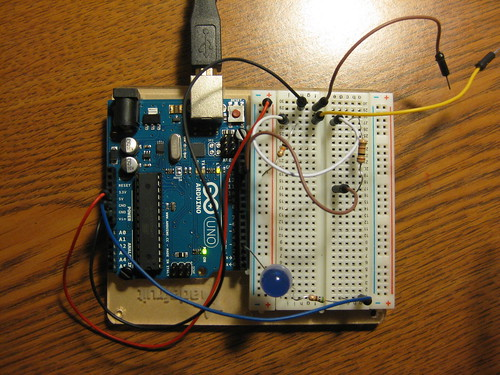# Help with millis...or atleast i think

Hello everyone!

I'm new to arduino so I'm hoping I can get some advice.

currently i'm running a loop with a button that i've replaced with wires i can touch together to close the "button," thus allowing the led to fade.what i want to figure out is how can i put a timer/millis (or anything that will make it work) into the loop so the first loop would be, when the wires touch, to "fade." then over time, like 5 seconds, have a second loop start with a more intense fade, then a third loop with a more intense fade and so on and so for. and by intense i mean to decrease the fade's delay.

the problem is i can't fit in what i've found regarding millis (A) into what i've programmed so far (B).

what i've found may be n/a but its what i've been working with.

hope i can get some long lasting advice.

thanks!

(A)

unsigned long startTime = 0;

void setup()
{
Serial.begin(9600);
}

void loop()
{
unsigned long loopTime = millis() - startTime; //Calculate the time since last time the cycle was completed

if (loopTime <= 1000) //Check if the time is less than 1000 millis, and if so, run loop 1
{
Serial.println("Loop 1");
}
else if (loopTime > 1000 && loopTime <= 2000) //If time is over 1000 millis and less than/or 2000 millis, run loop 2
{
Serial.println("Loop 2");
}
else if (loopTime > 2000) //If time is over 2000 millis, set the startTime to millis so the loop time will be reset to zero
{
startTime = millis();
}

delay(250); //Only here to prevent spamming serial monitor too much
}

(B)

int ledPin = 3;
int switchPin = 13;
int val;
int brightness = 150;

void setup() {
pinMode(ledPin, OUTPUT);
pinMode(switchPin, INPUT);
}

void loop(){
if (val == LOW) {
analogWrite(ledPin, brightness);
if (brightness > 255) {
brightness = 0;
}
delay (150);
}
if (val == LOW) {
analogWrite(ledPin, brightness);
if (brightness > 255) {
brightness = 0;
}
delay (150);
}
}

You need two values - the time between fade steps changing and the amount to change the fade a\value by.

Over time, based on millis(), change the amount to fade by.

Separately, use millis() to determine if it is time to apply that fade amount and apply the new values to the pins.

I'm not quite sure i follow you,

would i need to add int?

like

int millisChange =
int millisValue =

...not sure what they would equal

You need to add several variables. You need one to hold the last time the fade amount changed. You need one to define how often the fade amount should change. You need one to hold the fade amount.

You need one to hold when the fade amount was last applied. You need one to define how often the fade amount should be applied. And, you need one to hold the current fade value.

Each time through loop, you need to get the current time. Then, see if it is time to change the fade amount. If so, change it, and record when you did that.

Separately, you need to see if it is time to change the fade value. If it is, change the fade value (by adding or subtracting the fade amount), apply it to the pins, and record when you did that.